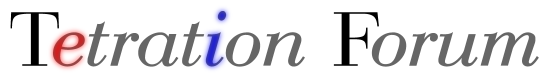Bivariate quasi-holomorphic function T(x,y) for complex x and y - Printable Version +- Tetration Forum (https://tetrationforum.org) +-- Forum: Tetration and Related Topics (https://tetrationforum.org/forumdisplay.php?fid=1) +--- Forum: Mathematical and General Discussion (https://tetrationforum.org/forumdisplay.php?fid=3) +--- Thread: Bivariate quasi-holomorphic function T(x,y) for complex x and y (/showthread.php?tid=509) Bivariate quasi-holomorphic function T(x,y) for complex x and y - Ciera_ΩMega - 09/04/2010 Consider a bivariate function T(x,y) for complex x and y that satisfies the following ① T(x,0) = 1 for x≠0 and abs(x)≠1 ② T(x,y+1) = x^T(x,y) ③ For constant x, x≠0 and abs(x)≠1, T(x,y) is bounded on the strip with Re(y)∈[0,1) and Im(y)∈(-∞,∞) ④ For constant real y, y≥0, Re(x)=0 and Im(x)≠0, Re(T(x,y)) and T(x,y)/x are bounded ⑤ T(x,y) is a function of two complex variables holomorphic outside from singularities and branch cuts Conditions ① and ② are basic conditions for tetration; ③ ensures that base-x tetrational cannot diverge for extreme values of Im(y), much as exp(y) is bounded for constant Re(y) and variable Im(y); ④ is derived from the fact that for Re(x)=0 and Im(y)=0, the iterated exponential of x as x→∞ⅈ and y→∞, approaches the 3-cycle {x, 0, 1}, which clearly has Re(T(x,y)) bounded and T(x,y)/x also bounded, and ⑤ ensures smoothness and analyticity of T(x,y) except on cuts and at singularities. The idea for a function described above comes from the thread (tid=380) about base holomorphic tetration for fixed height, and (tid=377) which describes tetration of complex bases to complex heights. How about a bivariate function that is quasi-holomorphic on both base and height, much like how complex bases can be raised to complex powers with quasi-holomorphism on base and exponent? What ideas can be made about uniqueness conditions for such a bivariate function? RE: Bivariate quasi-holomorphic function T(x,y) for complex x and y - bo198214 - 09/07/2010 Hey Ciera, welcome on board, before my retreat, some points about your post: I tried a bit about this "boundedness criterion" whether quasi-holomorphic or only holomorphic in the second variable. However I couldnt find a uniqueness criterion (for general functions not only exponentiation though). Such a criterion would be of great value, because it would unite the case be^(1/e) because it is applicable for both. I can give you only the two references containing uniqueness criterion for the case with real fixed point: http://citeseerx.ist.psu.edu/viewdoc/summary?doi=10.1.1.154.3249 and the case of two complex fixed points: http://arxiv.org/abs/1006.3981 also Mike3 posted lately a nice uniqueness criterion for regular iteration at a real fixed point. I am not sure whether considering the bivariate holomorphism really helps for uniqueness. But I wish you luck in proving such a criterion, really! (Dont forget however to make sure that your uniqueness criterion can be satisfied by any function at all!) RE: Bivariate quasi-holomorphic function T(x,y) for complex x and y - tommy1729 - 09/07/2010 (09/07/2010, 03:58 PM)bo198214 Wrote: also Mike3 posted lately a nice uniqueness criterion for regular iteration at a real fixed point. what exactly do you mean ? RE: Bivariate quasi-holomorphic function T(x,y) for complex x and y - bo198214 - 09/08/2010 (09/07/2010, 07:50 PM)tommy1729 Wrote: (09/07/2010, 03:58 PM)bo198214 Wrote: also Mike3 posted lately a nice uniqueness criterion for regular iteration at a real fixed point. what exactly do you mean ? I mean the thread Carlson's theorem and tetration. RE: Bivariate quasi-holomorphic function T(x,y) for complex x and y - mike3 - 09/08/2010 (09/04/2010, 05:25 PM)Ciera_ΩMega Wrote: Consider a bivariate function T(x,y) for complex x and y that satisfies the following ① T(x,0) = 1 for x≠0 and abs(x)≠1 ② T(x+1,y) = x^T(x,y) ③ For constant x, x≠0 and abs(x)≠1, T(x,y) is bounded on the strip with Re(y)∈[0,1) and Im(y)∈(-∞,∞) ④ For constant real y, y≥0, Re(x)=0 and Im(x)≠0, Re(T(x,y)) and T(x,y)/x are bounded ⑤ T(x,y) is a function of two complex variables holomorphic outside from singularities and branch cuts Conditions ① and ② are basic conditions for tetration; ③ ensures that base-x tetrational cannot diverge for extreme values of Im(y), much as exp(y) is bounded for constant Re(y) and variable Im(y); ④ is derived from the fact that for Re(x)=0 and Im(y)=0, the iterated exponential of x as x→∞ⅈ and y→∞, approaches the 3-cycle {x, 0, 1}, which clearly has Re(T(x,y)) bounded and T(x,y)/x also bounded, and ⑤ ensures smoothness and analyticity of T(x,y) except on cuts and at singularities. The idea for a function described above comes from the thread (tid=380) about base holomorphic tetration for fixed height, and (tid=377) which describes tetration of complex bases to complex heights. How about a bivariate function that is quasi-holomorphic on both base and height, much like how complex bases can be raised to complex powers with quasi-holomorphism on base and exponent? What ideas can be made about uniqueness conditions for such a bivariate function? I am a bit skeptical of condition 4, because I tried a construction once for a tetrational extension of such a "period" base, giving unbounded results at the real axis: http://math.eretrandre.org/tetrationforum/showthread.php?tid=437 and a graph in the height plane (what you call "y"-plane) is given on page 2. Note that the constructed function meets 1, 2, and 3, but not 4. Indeed, I'm not sure if we can have 4, without perhaps also losing 5. Condition 3 reminds me of Kouznetsov. Also, if it can be made to hold, it would seem to suggest that for complex bases inside the "Shell-Thron region" (the region where the tetrational converges to a fixed point at real heights approaching real infinity), there is an alternate solution besides regular iteration that might be the better one (because the regular has singularities in that strip and so cannot be bounded there).